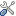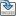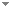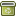# Proof Compression and NP Versus PSPACE II

Bulletin of the Section of Logic 49 (3):213-230 (2020)

 Authors Edward Haeusler Pontifícia Universidade Católica do Rio de Janeiro Abstract We upgrade  to a complete proof of the conjecture NP = PSPACE that is known as one of the fundamental open problems in the mathematical theory of computational complexity; this proof is based on . Since minimal propositional logic is known to be PSPACE complete, while PSPACE to include NP, it suﬃces to show that every valid purely implicational formula ρ has a proof whose weight and time complexity of the provability involved are both polynomial in the weight of ρ. As is , we use proof theoretic approach. Recall that in  we considered any valid ρ in question that had a “short” tree-like proof π in the Hudelmaier-style cutfree sequent calculus for minimal logic. The “shortness” means that the height of π and the total weight of diﬀerent formulas occurring in it are both polynomial in the weight of ρ. However, the size, and hence also the weight, of π could be exponential in that of ρ. To overcome this trouble we embedded π into Prawitz’s proof system of natural deductions containing single formulas, instead of sequents. As in π, the height and the total weight of diﬀerent formulas of the resulting tree-like natural deduction ∂1 were polynomial, although the size of ∂1 still could be exponential, in the weight of ρ. In our next, crucial move, ∂1 was deterministically compressed into a “small”, although multipremise, dag-like deduction ∂ whose horizontal levels contained only mutually diﬀerent formulas, which made the whole weight polynomial in that of ρ. However, ∂ required a more complicated veriﬁcation of the underlying provability of ρ. In this paper we present a nondeterministic compression of ∂ into a desired standard dag-like deduction ∂0 that deterministically proves ρ in time and space polynomial in the weight of ρ.2 Together with  this completes the proof of NP = PSPACE.Natural deductions are essential for our proof. Tree-to-dag horizontal compression of π merging equal sequents, instead of formulas, is not suﬃcient, since the total number of diﬀerent sequents in π might be exponential in the weight of ρ – even assuming that all formulas occurring in sequents are subformulas of ρ. On the other hand, we need Hudelmaier’s cutfree sequent calculus in order to control both the height and total weight of diﬀerent formulas of the initial tree-like proof π, since standard Prawitz’s normalization although providing natural deductions with the subformula property does not preserve polynomial heights. It is not clear yet if we can omit references to π even in the proof of the weaker result NP = coNP. Keywords No keywords specified (fix it) Categories (categorize this paper) DOI 10.18778/0138-0680.2020.16 OptionsEdit this recordMark as duplicateExport citationFind it on ScholarRequest removal from indexRevision history

PhilArchive copy

Upload a copy of this paper     Check publisher's policy     Papers currently archived: 69,160

Setup an account with your affiliations in order to access resources via your University's proxy server
Configure custom proxy (use this if your affiliation does not provide a proxy)

## References found in this work BETA

Embedding Classical in Minimal Implicational Logic.Hajime Ishihara & Helmut Schwichtenberg - 2016 - Mathematical Logic Quarterly 62 (1-2):94-101.

## Citations of this work BETA

No citations found.

## Similar books and articles

Propositional Proof Compressions and DNF Logic.L. Gordeev, E. Haeusler & L. Pereira - 2011 - Logic Journal of the IGPL 19 (1):62-86.
Propositional Consistency Proofs.Samuel R. Buss - 1991 - Annals of Pure and Applied Logic 52 (1-2):3-29.
A Note on Propositional Proof Complexity of Some Ramsey-Type Statements.Jan Krajíček - 2011 - Archive for Mathematical Logic 50 (1-2):245-255.
Examining Fragments of the Quantified Propositional Calculus.Steven Perron - 2008 - Journal of Symbolic Logic 73 (3):1051-1080.
Complexity, Decidability and Completeness.Douglas Cenzer & Jeffrey B. Remmel - 2006 - Journal of Symbolic Logic 71 (2):399 - 424.
Sharpened Lower Bounds for Cut Elimination.Samuel R. Buss - 2012 - Journal of Symbolic Logic 77 (2):656-668.
Frege Proof System and TNC°.Gaisi Takeuti - 1998 - Journal of Symbolic Logic 63 (2):709 - 738.
Frege Proof System and TNC$^Circ$.Gaisi Takeuti - 1998 - Journal of Symbolic Logic 63 (2):709-738.